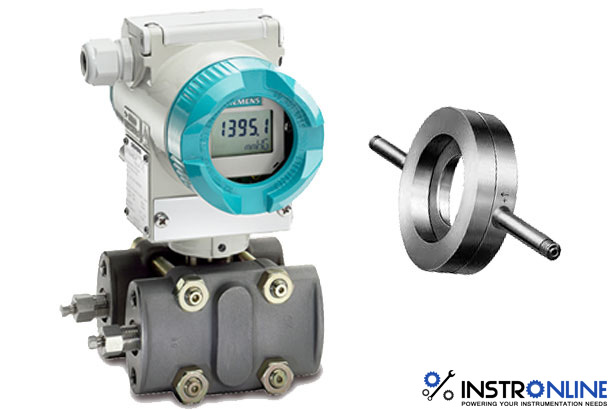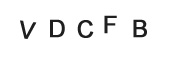• Home
• »
• Blog
• »
• What is a Differential Pressure Flow meter?
Discussion Forum

##### What is a Differential Pressure Flow meter?
Listed on Feb 28,2019Differential pressure meters work on the principle of partially obstructing the flow in a pipe. This creates a difference in the static pressure between the upstream and downstream side of the device. This difference in the static pressure (referred to as the differential pressure) is measured and used to determine the flowrate.

Differential-pressure meters are hugely popular and it is estimated that at least 40% of industrial flow meters in use at present are differential-pressure devices, with the orifice plate being the most popular. Differential-pressure devices have been used to meter a wide variety of different fluids from gases to highly viscous liquids.

The popularity of differential-pressure flow meters is in part due to their simple design and low cost. By reading this guide you will have a much clearer idea of the benefits, viable metering options and applications for using differential-pressure meters.

How a Δp flow meters works

The concept of using the pressure drop caused by a fluid flowing through a restriction in a pipe as a measurement of flow rate dates back to the 18th Century, when it was discovered by Bernoulli.

The basic principle of how a Δp flowmeter operates is described in the figure below.

The differential pressure principle. Manometer tubes measure the difference in static pressure upstream and downstream of the restriction

When a fluid flows through a restriction, it accelerates to a higher velocity (i.e. V2 > V1 ) to conserve the mass flow and, as a consequence of this, its static pressure drops. This differential pressure (Δp) is then a measure of the flowrate through the device.

In simple terms for a given size of restriction, the higher the Δp, the higher the flowrate.

The relationship between the differential pressure and flowrate is derived from Bernoulli’s equation. Using Bernoulli’s equation, and conservation of mass , it can be shown that the differential pressure generated is proportional to the square of the mass flowrate, Qm (kg/s)

Many of the Δp meters available work on this principle of measuring the difference in pressure between upstream and downstream but there are some meters which use the differential pressure in other ways, for example, variable area meters.

What are the different types of Δp meter?

The most common types of differential pressure meter are:
• Orifice plates
• Venturi tubes
• Cone meters (e.g. V-cones)
• Nozzles
• Low loss meters (e.g. Dall tubes)
• Variable area meters
• Inlet flow meters
• Venturi cones
• Venturi nozzles
• Drag plates

There are a number of general advantages common to most Δp meters. These include:

• They are simple to make, containing no moving parts

• Their performance is well understood

• They are cheap – especially in larger pipes when compared with other meters

• They can be used in any orientation

• They can be used for most gases and liquids

• Some types do not require calibration for certain applications

The main disadvantages to Δp meters are:

• Rangeability (turndown1 ) is less than for most other types of flowmeter

• Significant pressure losses may occur

• The output signal is non-linear with flow

• The discharge coefficient and accuracy may be affected by pipe layout or nature of flow

• They may suffer from ageing effects, e.g. the build-up of deposits or erosion of sharp edges

Common terminology

The diameter ratio or beta (sometimes referred to as the beta ratio) is the ratio between the diameter of the orifice or throat of device to that of the pipe.

Often Δp meters are described in terms of their beta value and diameter to fit a certain pipeline size, for example, a 4-inch β= 0.6 Venturi tube.

To state that a Δp meter has a low beta ratio, for example β = 0.2, means the plate has a small hole or restriction size. This causes the pressure loss across the Δp meter to be higher, which may mean that a pump with a higher discharge pressure (hence more expensive) or compressor will be needed to overcome the increased pressure loss and maintain a flowrate achievable with a larger beta Δp meter. On the other hand a higher differential pressure can generally be measured more accurately than a lower one.

Effect of using different values of beta

The effect of using larger values of beta include:
• an increase in the discharge coefficient uncertainty
• a lower differential pressure being measured across the orifice plate (and this can be more difficult to measure)
• longer lengths of upstream straight pipe being required to ensure the velocity profile of the flow through the orifice plate is stable and symmetrical
• the flow profile of the flow through the orifice being more affected by the roughness of the pipe walls
There are a number of sizing packages for orifice plates available, which will calculate the dimensions of the plate required. The software uses empirical formulae based on actual testing. Most of the results are available for beta values of 0.3 to 0.7.

• Low cost
• Ease of installation
• Availability of comprehensive standard (ISO 5167-2)
• No requirement for calibration – value of C from the standard
• Availability of different designs, e.g. for viscous fluids, bi-directional flows, suspended solids
• Low turn down (can be improved with dual range Δp cells)
• High pressure loss (35 to almost 100% of measured Δp depending on beta)
• Errors due to erosion / damage to upstream edges
• Errors due to high sensitivity to upstream installation (especially large beta devices)
Discharge coefficient (C)

The discharge coefficient, C, is a parameter that takes account of non-ideal effects, for example energy losses due to friction, when using Δp meters. The discharge coefficient is basically the ratio of the actual to the measured mass flowrate.

The discharge coefficient can either be:

1. determined from a standard
• provides good flow measurement at a reasonable price
• is especially suitable where repeatability is more important than accuracy
or

2. determined by calibration
• provides lower uncertainties on the flow measurement.
In nozzles and Venturi tubes the flow follows the boundary of the tube closely and the value of C is usually close to one. However, for orifice plates C has a value of approximately 0.6. Values of C can be obtained from the standard (ISO 5167) for nozzles, Venturi tubes and orifice plates that are manufactured to the specified tolerances of the standard.Tagged Popular Searches

Related Search